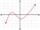# Quadratic function 2

Which of the points belong function f:y= 2x2- 3x + 1 :

A(-2, 15)
B (3,10)
C (1,4)

Result

A=

B=

C=

#### Solution:Leave us a comment of example and its solution (i.e. if it is still somewhat unclear...):

Showing 0 comments:Be the first to comment!#### To solve this example are needed these knowledge from mathematics:

Looking for help with calculating roots of a quadratic equation?

## Next similar examples:

1. Quadratic functionWrite the equation of the quadratic function which includes points A (-1, 10), B (2, 19), C (1,4)
2. Expressions 3If k(x+6)= 4x2 + 20, what is k(10)=?
3. EquationEequation f(x) = 0 has roots x1 = 64, x2 = 100, x3 = 25, x4 = 49. How many roots have equation f(x2) = 0 ?
4. Quadratic equationFind the roots of the quadratic equation: 3x2-4x + (-4) = 0.
5. RootsDetermine the quadratic equation absolute coefficient q, that the equation has a real double root and the root x calculate: ?
6. EquationEquation ? has one root x1 = 8. Determine the coefficient b and the second root x2.
7. DiscriminantDetermine the discriminant of the equation: ?
8. CombinationsHow many elements can form six times more combinations fourth class than combination of the second class?
9. Solve 3Solve quadratic equation: (6n+1) (4n-1) = 3n2
10. CombinationsFrom how many elements we can create 990 combinations 2nd class without repeating?
11. Variations 4/2Determine the number of items when the count of variations of fourth class without repeating is 600 times larger than the count of variations of second class without repetition.
12. Quadratic equationSolve quadratic equation: 2x2-58x+396=0
13. Expression with powersIf x-1/x=5, find the value of x4+1/x4
14. ProductThe product of two consecutive odd numbers is 8463. What are this numbers?
15. AlgebraX+y=5, find xy (find the product of x and y if x+y = 5)
16. AsymptoteWhat is the vertical asymptote of ?
17. Theorem proveWe want to prove the sentense: If the natural number n is divisible by six, then n is divisible by three. From what assumption we started?